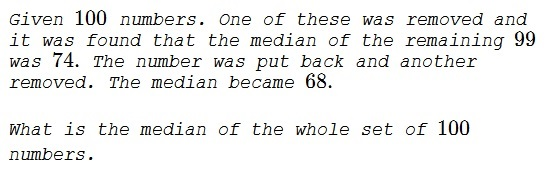# A Question about the Median

### Problem### Solution 1

Place the numbers in increasing order, say, $a_1\le a_2\le\ldots\le a_{100}.$ The median of the set is $\displaystyle \frac{a_{50}+a_{51}}{2}.$

If a number, say $a_j,$ was removed with $j\le 50$ then $a_{51}$ becomes the median; with $j\gt 50,$ $a_{50}$ becomes a median. It follows that $a_{50}=68$ and $a_{51}=74.$

The median of all $100$ numbers is then $\displaystyle \frac{68+74}{2}=71.$

### Solution 2

Let $S=\{a_1,a_2,\ldots,a_{50},a_{51},\ldots,a_{100}\},$ arranged by rank. Let $M(T)$ be the median of set $T.$ Note that the removal of any term above the median has the same effect; same as below. It follows that in the two experiments the items have been removed from different halves of the set $S.$

$\displaystyle M(S\setminus \{a_{\gt 50}\}=a_{50}=68,\\ M(S\setminus \{a_{\le 50}\}=a_{51}=74.$

The median $M(S)$ is therefore $71.$

### Acknowledgment

This is a paraphrase of problem 65312 from a Russian problem collection. Solution 2 is by N. N. Taleb.